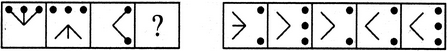# Non Verbal Reasoning - Analogy - Discussion

### Discussion :: Analogy - Section 1 (Q.No.5)

Each of the following questions consists of two sets of figures. Figures A, B, C and D constitute the Problem Set while figures 1, 2, 3, 4 and 5 constitute the Answer Set. There is a definite relationship between figures A and B. Establish a similar relationship between figures C and D by selecting a suitable figure from the Answer Set that would replace the question mark (?) in fig. (D).

5.

Select a suitable figure from the Answer Figures that would replace the question mark (?).(A)     (B)      (C)     (D)                  (1)      (2)      (3)      (4)      (5)

 [A]. 1 [B]. 2 [C]. 3 [D]. 4 [E]. 5

Explanation:

Except for the dots, the remaining part of the figure rotates through 180o and shifts to the opposite side of the square boundary.

 Pradeep said: (Nov 13, 2013) I guess it must be option 3 as the dots remain constant and remaining figure rotates opposite.

 Abc said: (Jan 18, 2017) Why not option 4?

 Vishwadeep said: (Jun 3, 2017) I think option D is the correct answer.

 Bhavna said: (Jun 24, 2018) According to me, Option D is the correct answer.

 Bhagavan Reddy said: (Mar 7, 2019) Option D would be the correct answer.

 Vansh Gurjar said: (Aug 4, 2019) According to me, option D is correct.

 Jagadeesh said: (Aug 12, 2020) Option D is correct.

 Babloo said: (May 18, 2021) Why 3 dots will be there?